2019.723，这个数的二进制形式是什么样呢？让我们慢慢考虑。

数字的记录

1 -> I

2 -> II

3 -> III

4 -> IIII

5 -> IIIII

20 -> ???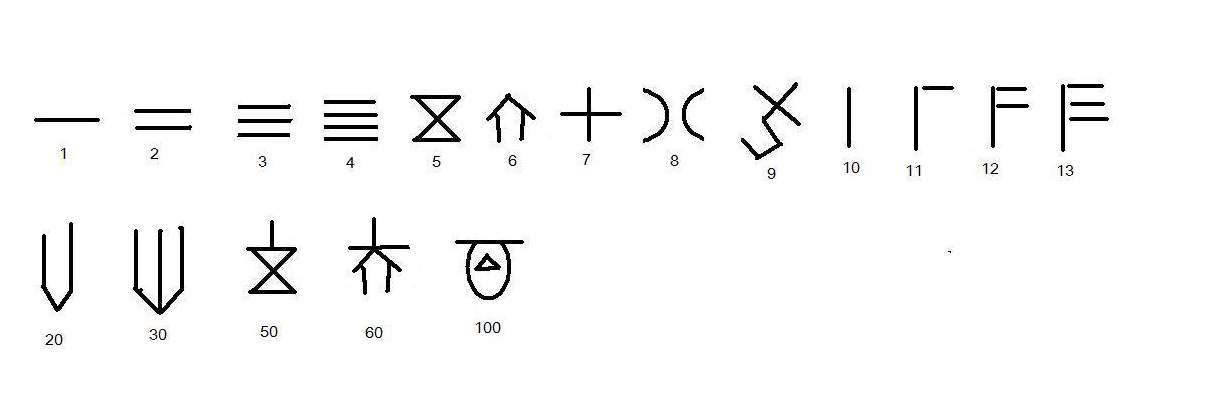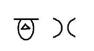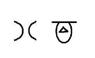进制的概念

$$…+a\times10^3+b\times10^2+c\times10^1+d\times10^0+e\times10^{-1}+f\times10^{-2}+g\times10^{-3}+…$$

a，b，c … 代表0 - 9中的任意一个符号，现在的数量是a1000，b 个 100，c 个 10……

$$2\times10^3+0\times10^2+1\times10^1+9\times10^0+7\times10^{-1}+2\times10^{-2}+3\times10^{-3}$$

2 进制只有两个符号可以用，那就是01，规则是满2 就要进 1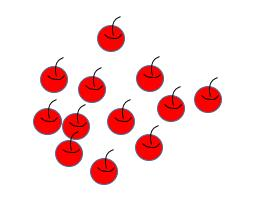二进制转十进制

$$…+a\times2^3+b\times2^2+c\times2^1+d\times2^0+e\times2^{-1}+f\times2^{-2}+g\times2^{-3}+…$$

a，b，c … 代表01中的一个，现在的数量是 a 个 8，b 个 4，c 个 2 ……

十进制转二进制

整数的转换

$$…a\times2^4+b\times2^3+c\times2^2+d\times2^1+e\times2^0=2019$$

$$…a\times2^3+b\times2^2+c\times2^1+d\times2^0+e/2=2018/2+1/2$$

$$e/2==1/2$$

$$…a\times2^3+b\times2^2+c\times2^1+d\times2^0=2018/2=1009$$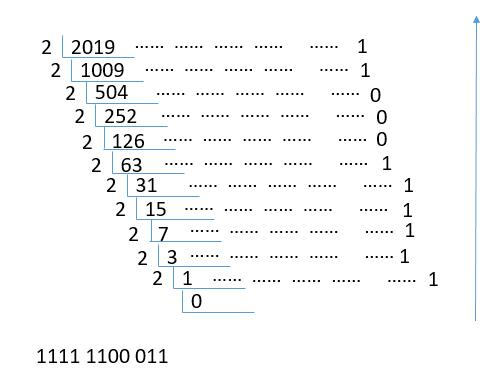小数的转换

$$e\times2^{-1}+f\times2^{-2}+g\times2^{-3}+…=0.723$$

$$e\times2^{0}+f\times2^{-1}+g\times2^{-2}+…=0.723\times2$$

$$e+f\times2^{-1}+g\times2^{-2}+…=1+0.446$$

$$e==1$$

$$f\times2^{-1}+g\times2^{-2}+…=0.446$$

$$f+g\times2^{-1}+…=0 + 0.892$$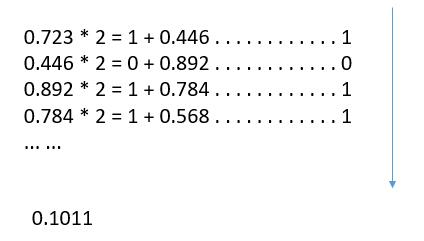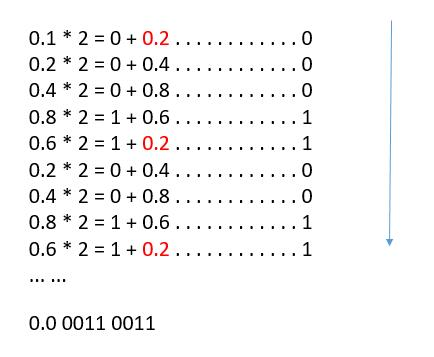历史的发展

• 古埃及十进制：以一个竖道代表1，二并排竖道代表2，三竖道代表3，一横道代表4，左二撇右竖道代表5，上三撇下三撇代表6，上下两道代表8，四个（并排代表9，一个“人”字形代表10，“人”上加一横代表20，20左加一点代表30，横道上加一点代表40，横道上加三竖道（如中国筹算的8）代表60，横道上加四竖道代表80（形同中国筹算中的9）代表80，两横道上加三竖代表90……。
• 古希腊十进制，1至9，10至90，100至900各有不同的单字母代表。
• 古印度Kharosshi十进制，以一个竖道代表1，二并排竖道代表2，三竖道代表3，一个X代表4，IX代表5，||X代表6，XX代表8，10，20个有单字符代表。
• 古印度和Brahmi十进制，和希腊十进制相似，1至9，10至90，100至900各有不同的单字母代表。符号很多。

• 巴比伦60进位制：以一个上大下小的楔形代表1，两个并列楔形代表2，三个并列楔形代表3，上二个楔形下二个楔形代表4，上三楔下二楔代表5，上三楔下三楔代表6，上四楔下三楔代表7，上四楔下四楔代表8，上五楔下四楔代表9；一个左小右大横楔代10，两个横楔并排代表20，三个横楔并排代表30，四个横楔并排代表40。
• 玛雅20进位制：以一个点代表1，两个点并列代表2，三点并列代表3，四点并列代表4，短横线代表5，横线上加一点代表6，横线上加二点代表7，横线上加三点代表8，横线上加四点代表9；上下两横线代表10，上下两横线之上加一点代表11，三重叠横线代表15，三横线上加一，二，三点代表16，17，18；小椭圆圈上加一点代表20。

• 中国古代的十进制有书写式和算筹两种型式。
• 印度-阿拉伯十进位制。

结束